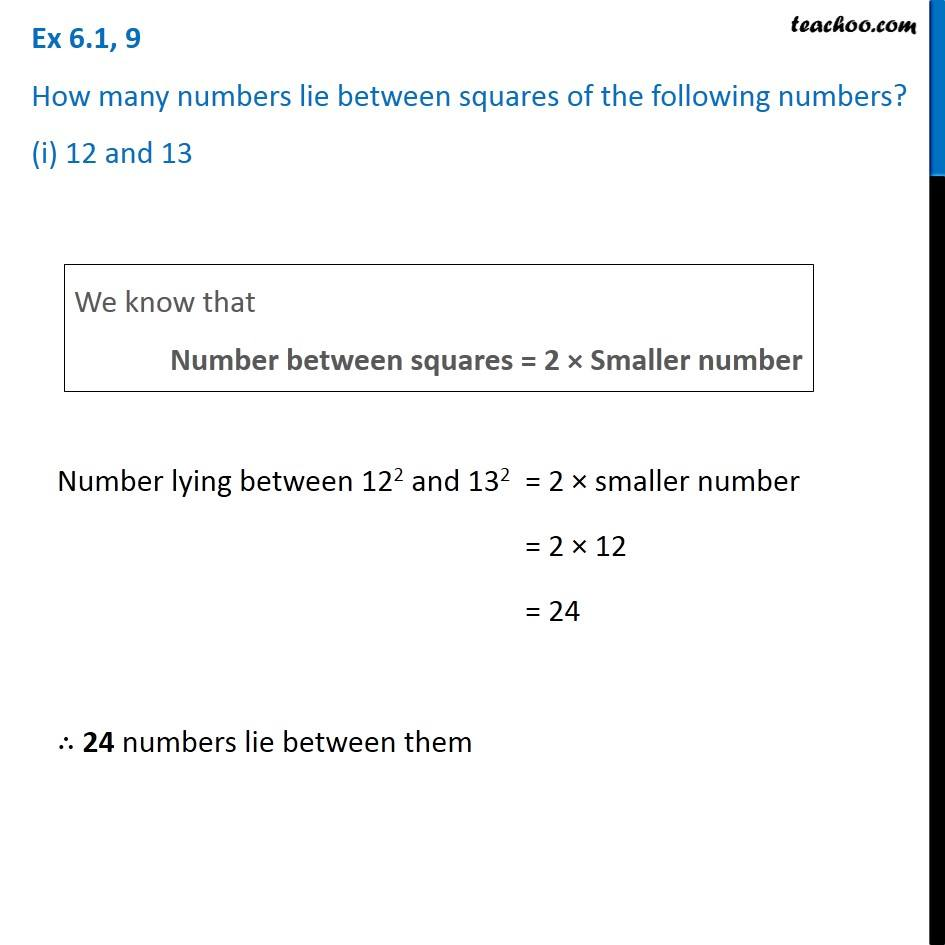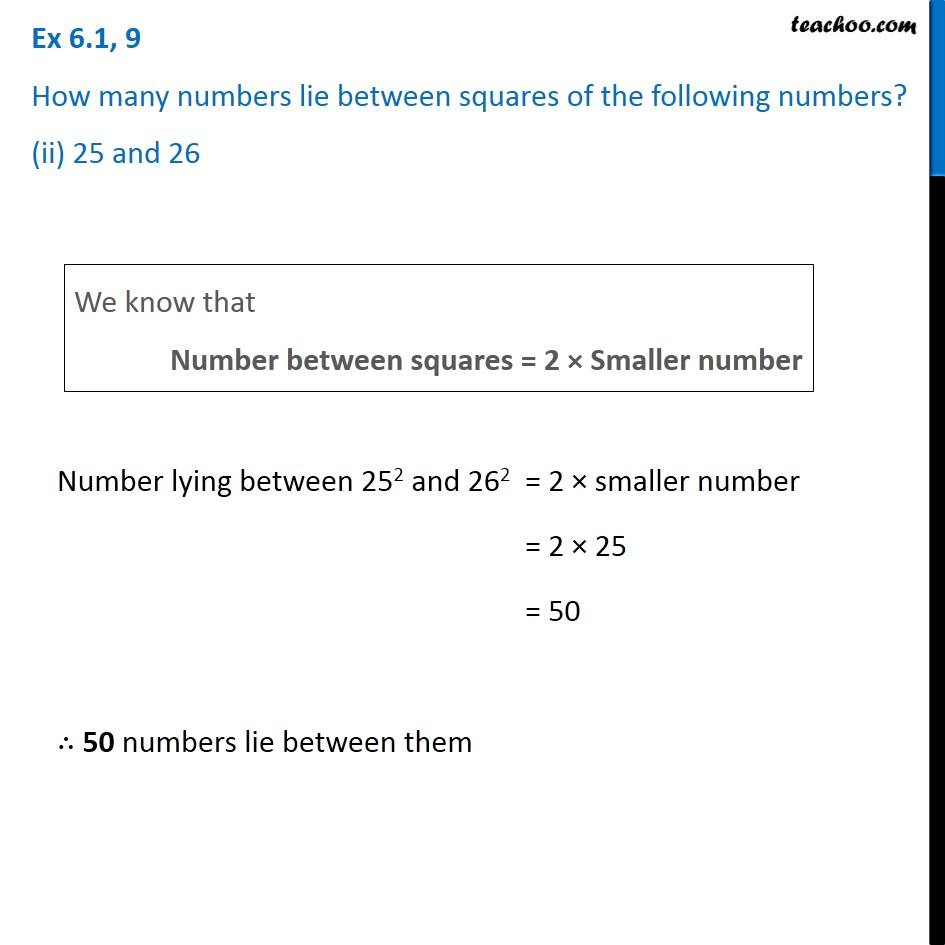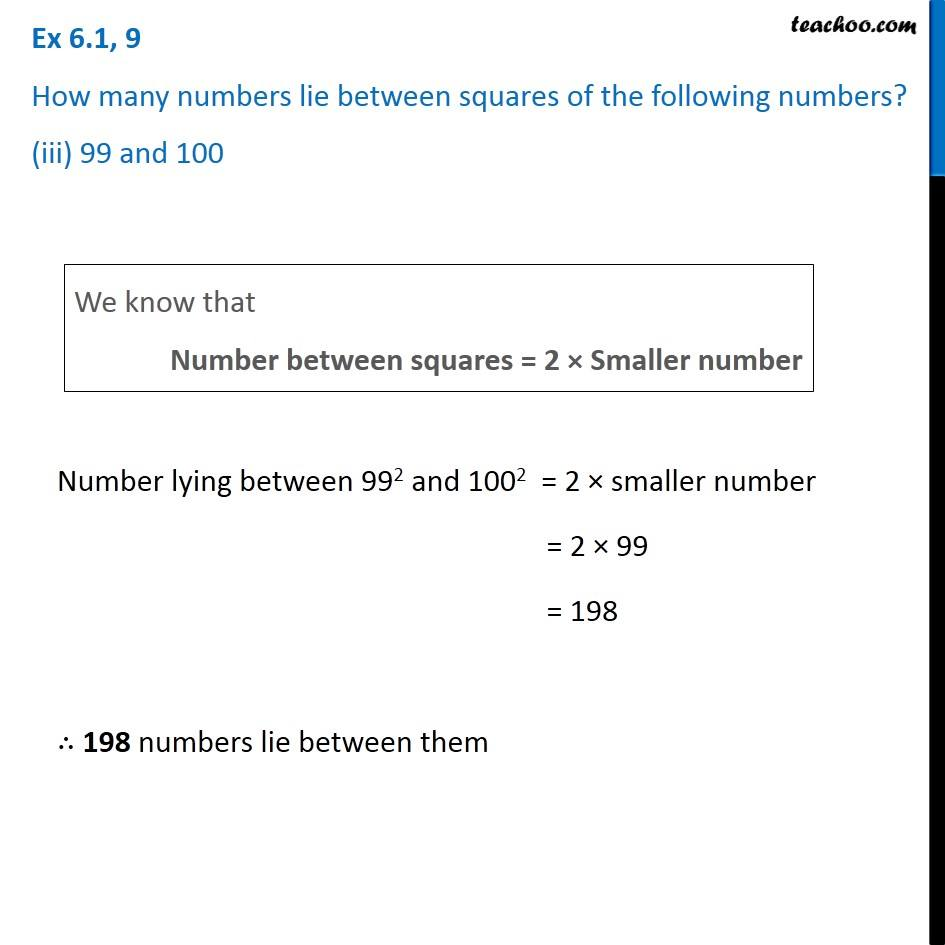1. Chapter 6 Class 8 Squares and Square Roots
2. Serial order wise
3. Ex 6.1

Transcript

Ex 6.1, 9 How many numbers lie between squares of the following numbers? (i) 12 and 13We know that Number between squares = 2 × Smaller number Number lying between 122 and 132 = 2 × smaller number = 2 × 12 = 24 ∴ 24 numbers lie between them Ex 6.1, 9 How many numbers lie between squares of the following numbers? (ii) 25 and 26We know that Number between squares = 2 × Smaller number Number lying between 252 and 262 = 2 × smaller number = 2 × 25 = 50 ∴ 50 numbers lie between them Ex 6.1, 9 How many numbers lie between squares of the following numbers? (iii) 99 and 100We know that Number between squares = 2 × Smaller number Number lying between 992 and 1002 = 2 × smaller number = 2 × 99 = 198 ∴ 198 numbers lie between them

Ex 6.1